# Lesson 3 3 Slopes of Lines Transparency 3

• Slides: 20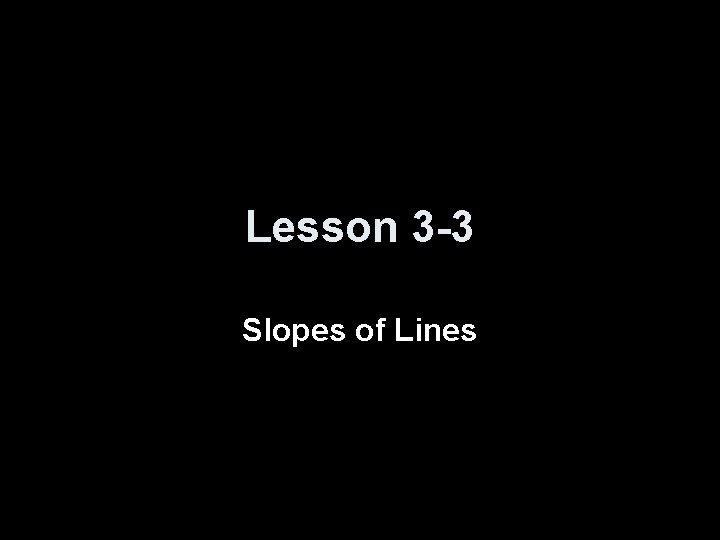Lesson 3 -3 Slopes of Lines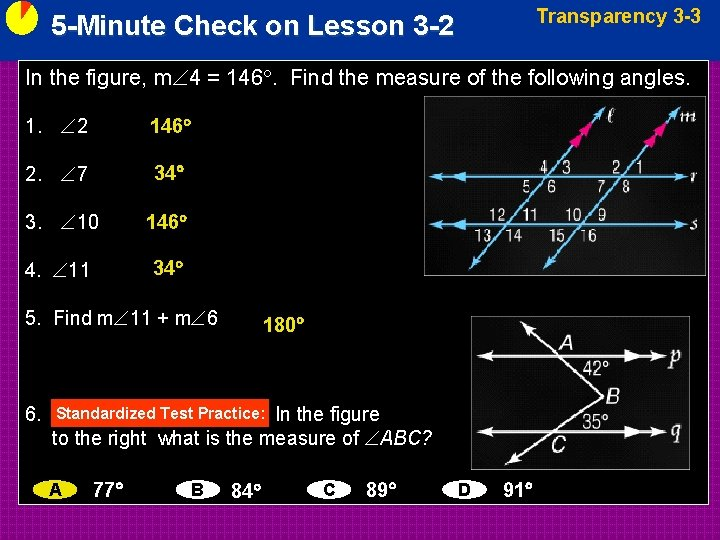Transparency 3 -3 5 -Minute Check on Lesson 3 -2 In the figure, m 4 = 146. Find the measure of the following angles. 1. 2 146 2. 7 34 3. 10 146 4. 11 34 5. Find m 11 + m 6 6. 180 In the figure to the right what is the measure of ABC? Standardized Test Practice: A 77 B 84 C 89 D 91Objectives • Find slopes of lines • Use slopes to identify parallel and perpendicular linesVocabulary • Slope – symbol m; the vertical rise divided by the horizontal run (or m = y/ x) A horizontal line has a slope of zero (no change in y; y = 0) A vertical line has an undefined slope (no change in x; x = 0) • Rate of change – describes how a quantity changes over time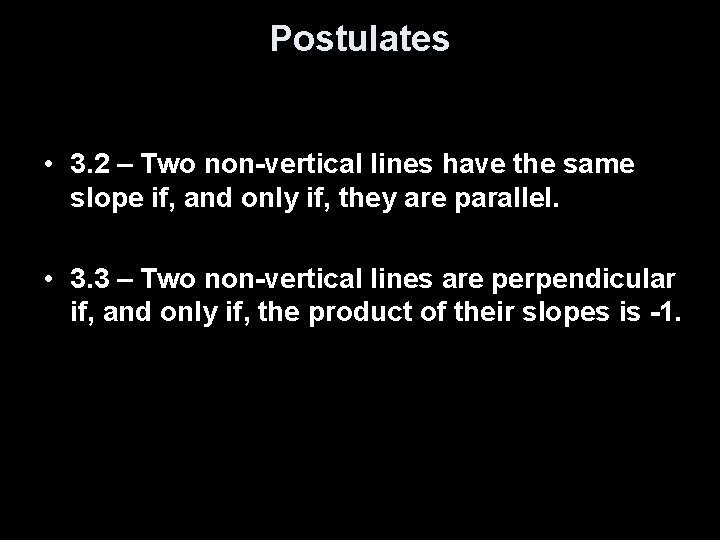Postulates • 3. 2 – Two non-vertical lines have the same slope if, and only if, they are parallel. • 3. 3 – Two non-vertical lines are perpendicular if, and only if, the product of their slopes is -1.Review of Slopes • Slope, m, is a rate of change (∆y / ∆x) between two points (x 1, y 1) and (x 2, y 2) y y 2 – y 1 ∆y m = -----x 2 – x 1 ∆x x • Two lines are parallel (never intersect), if they have the same slope (myellow = 1) • Two lines are perpendicular (forms a right angle), if their slopes multiply together to get -1 (mpink = -1)Find the slope of the line. From (– 3, 7) to (– 1, – 1), go down 8 units and right 2 units. Answer: – 4Find the slope of the line. Use the slope formula. Let be be . and Answer: undefined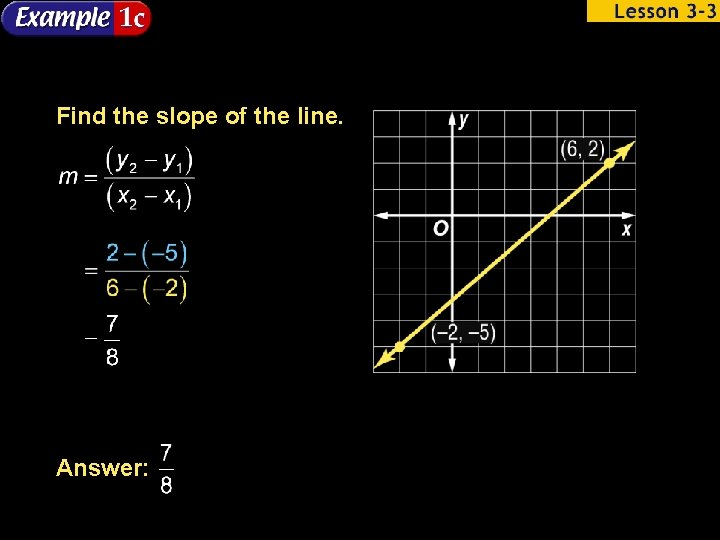Find the slope of the line. Answer: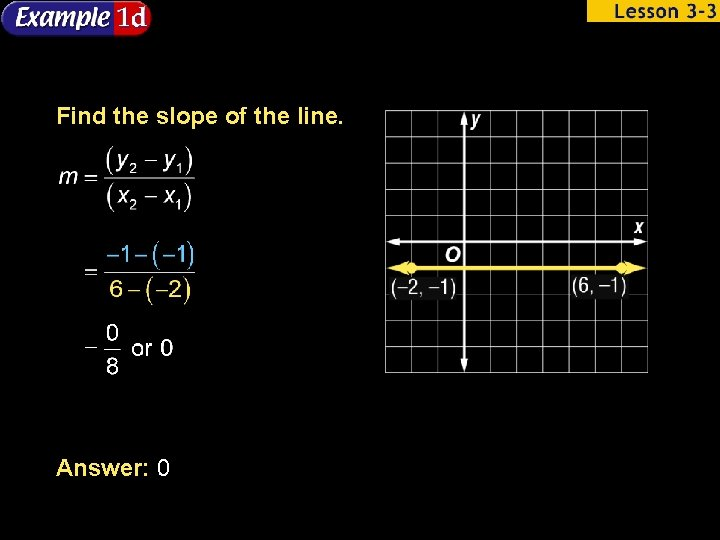Find the slope of the line. Answer: 0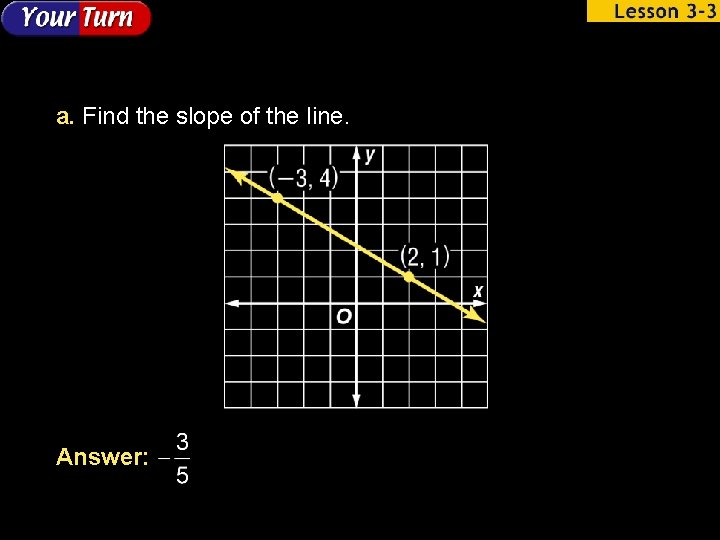a. Find the slope of the line. Answer:b. Find the slope of the line. Answer: 0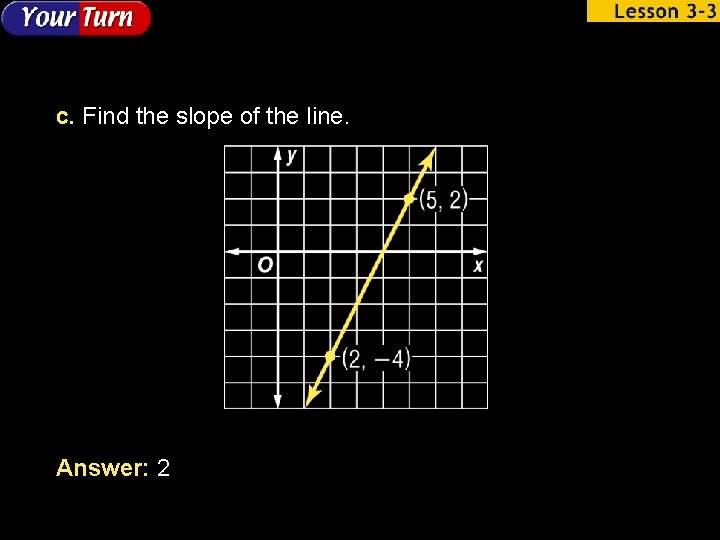c. Find the slope of the line. Answer: 2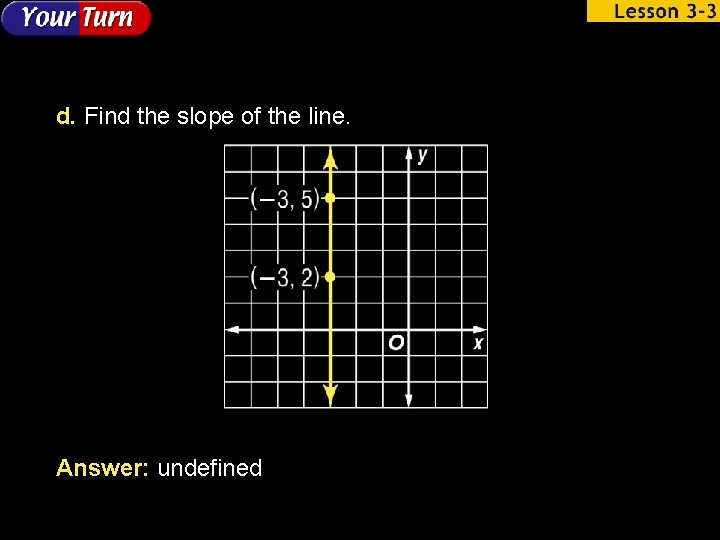d. Find the slope of the line. Answer: undefined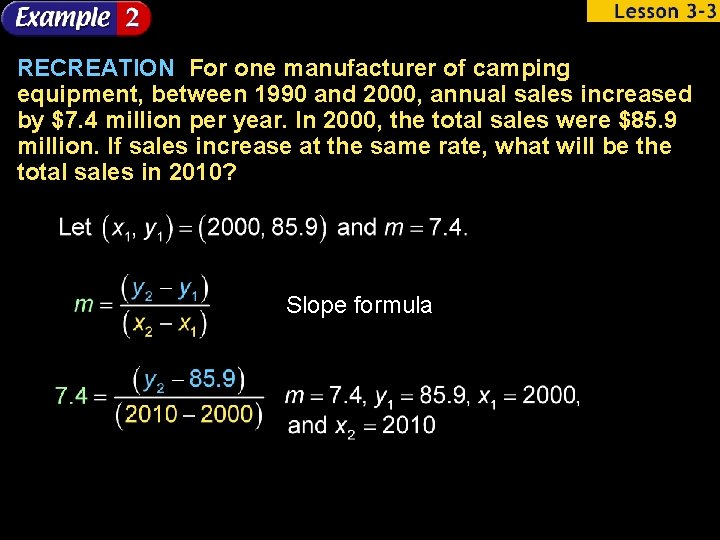RECREATION For one manufacturer of camping equipment, between 1990 and 2000, annual sales increased by \$7. 4 million per year. In 2000, the total sales were \$85. 9 million. If sales increase at the same rate, what will be the total sales in 2010? Slope formula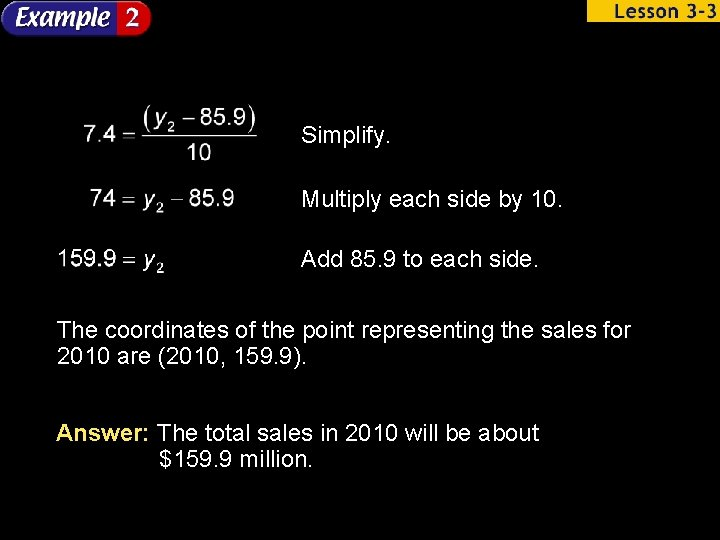Simplify. Multiply each side by 10. Add 85. 9 to each side. The coordinates of the point representing the sales for 2010 are (2010, 159. 9). Answer: The total sales in 2010 will be about \$159. 9 million.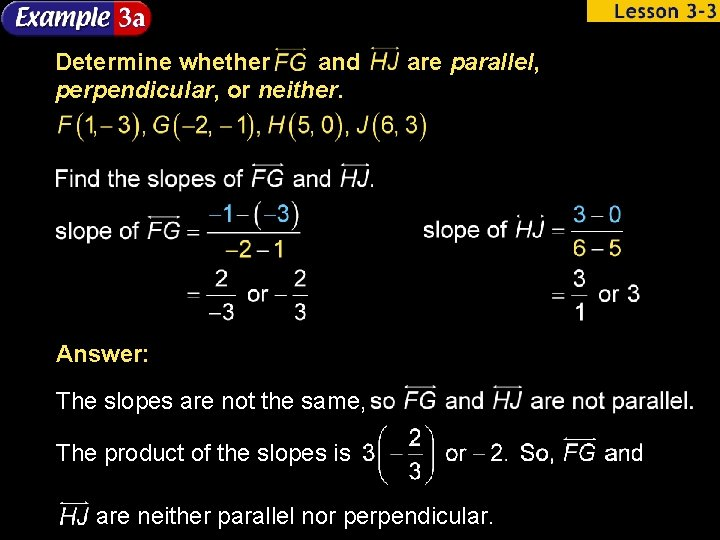Determine whether and perpendicular, or neither. are parallel, Answer: The slopes are not the same, The product of the slopes is are neither parallel nor perpendicular.Determine whether and perpendicular, or neither. are parallel, Answer: The slopes are the same, so are parallel.Determine whether and perpendicular, or neither. a. Answer: perpendicular b. Answer: neither are parallel,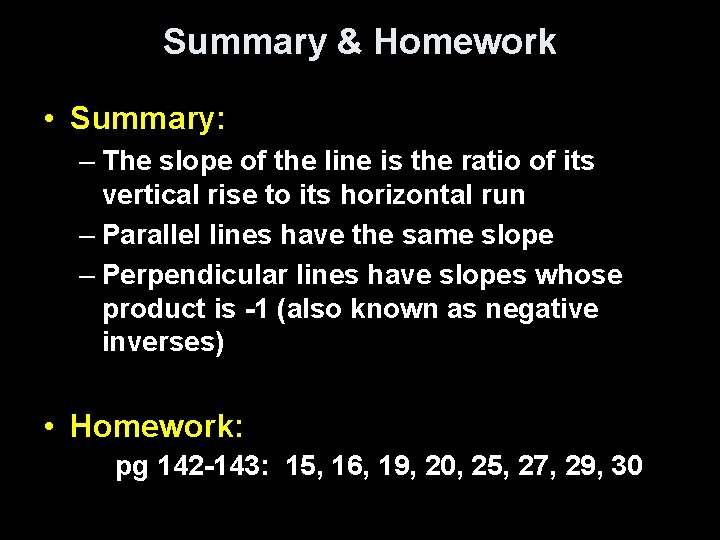Summary & Homework • Summary: – The slope of the line is the ratio of its vertical rise to its horizontal run – Parallel lines have the same slope – Perpendicular lines have slopes whose product is -1 (also known as negative inverses) • Homework: pg 142 -143: 15, 16, 19, 20, 25, 27, 29, 30